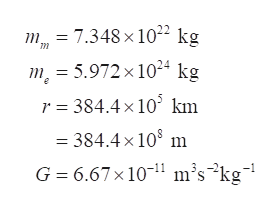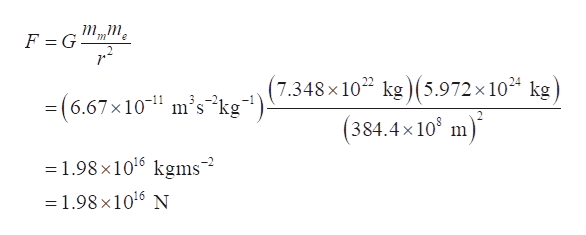The moon has a mass of 7.348 X 1022 kg and is 384.4 X 105 km from the Earth, which has a mass of 5.972 X 1024 kg. Solve for the force of gravity between the Earth and Moon. (ans: 1.98 X 1016 N) Show work.

Question

The moon has a mass of 7.348 X 1022 kg and is 384.4 X 105 km from the Earth, which has a mass of 5.972 X 1024 kg. Solve for the force of gravity between the Earth and Moon. (ans: 1.98 X 1016 N) Show work.

Step 1

Let mm be the mass of moon, me be the mass of earth, r be the distance between earth and moon, and G be the gravitational constant.

Write the given values of question.help_outlineImage Transcriptionclosem7.348 x1022 kg m = 5.972 x 1024 kg r 384.4x10 km = 384.4x 108 m G 6.67x 101 m's^kg1 fullscreen
Step 2

Write the expression for the gravitational force betwe...help_outlineImage Transcriptionclosee (7.348x 102 kg ) (5.972 x 104 kg 6.67x101 m's2kg° (384.4x10 m -1.98 x1016 kgms2 1.98 x 1016 N fullscreen

Want to see the full answer?

See Solution

Want to see this answer and more?

Our solutions are written by experts, many with advanced degrees, and available 24/7

See Solution
Tagged in

Gravitation